Fabric Weight Calculation in GSM | Textile Study Center

# Fabric Weight Calculation in GSM

47.24K
0#### Taukir Kabir Tusar## Fabric Weight Calculation in GSM (Woven Fabric)

GSM calculation of fabric

Fabric is the basic raw material to produce any particular garments . Before going to bulk production , it is quite essential to know and test the GSM of the supplied fabric by the supplier. This GSM can directly be got through  GSM cutter .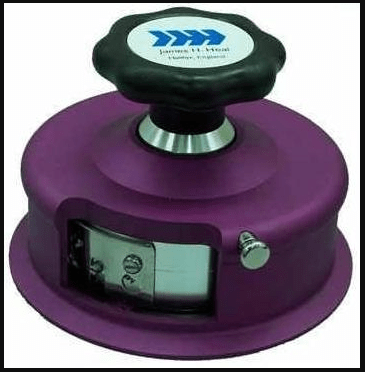But the GSM can be theoretically evaluated via some calculations . We will discuss here that process.

What is GSM?

Fabric GSM means the weight of fabric in grams in per square meter area. So the higher the GSM , the higher bulkier will be the fabric and vice versa , even they can be of same construction

Fabric Costruction

Fabric  Construction is a term in which some fabric detailing are available such as

EPI (Ends per inch –warp)

PPI (Picks per inch – weft)

Warp count

Weft count

fabric width (in inches) .

The formula :Some Examples :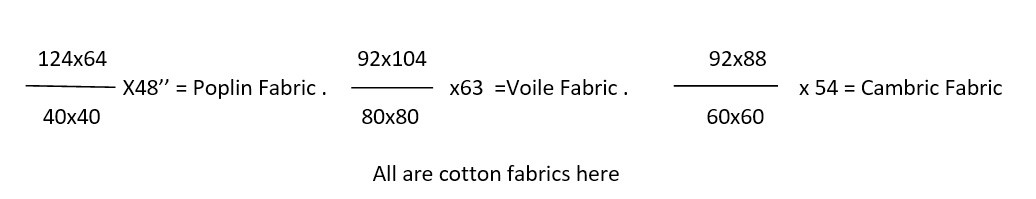Calculation of GSM :

We wil calculate GSM for  below one here –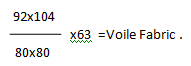Warp weight calculation :

Here ,       EPI = 92

So total number of ends = 92×39.37

= 3622.04

(as we have to go to meter cause EPI is in inches but GSM is in meter unit and 1 meter=39.37 inches )

Length of each 1 meter yarn allowing the crimp %  = 1m + 1m x 3%  ( Let, warp crimp = 3%)

= 1m + 1m x  0.03

= 1m + 0.03 m

= 1.03 m

(this is necessary to find out 1 meter yarn length because when we take 1 meter in both warp and weft , it’s covered area will be 1m x 1m =1 m which is identical to GSM unit that is – Grmas per Square Meter)

So . total length of warp yarn = 3622.04×1.03

= 3730.71 m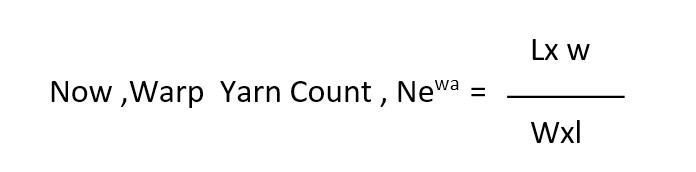( Here , L = Length found , w=1 lb  =  453.6 gm , W= Weight found/needed , l = 840×0.9144 meter)

[ As from the above formula , 453.6 gm means  1lb and 0 .9144 meter means 1 yd which came from the definition of Ne where weight unit is fixed to 1lb and length unit to 840 yds as a constant in the formula]

The number of hanks required of each having  840yds (1 hank)  of yarn that can make 1 lb weight is called the count (Ne) of that yarn.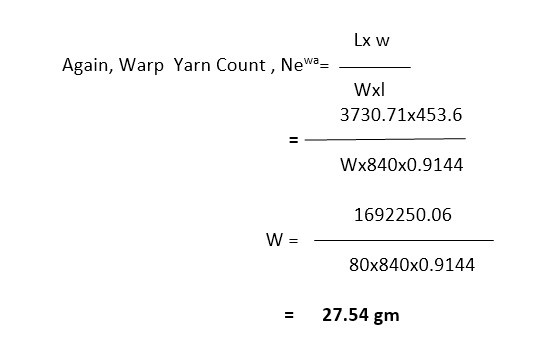Weft  weight calculation :

Here , PPI = 104

So total number of ends = 104×39.37 = 4094.48

Length of each 1 meter yarn allowing the crimp %  = 1m + 1m x 5%  ( Let, warp crimp = 5%)

= 1m + 1m x  0.05

= 1m + 0.05 m

= 1.05 m

So . total length of weft yarn = 4094.48×1.05

= 4299.21 m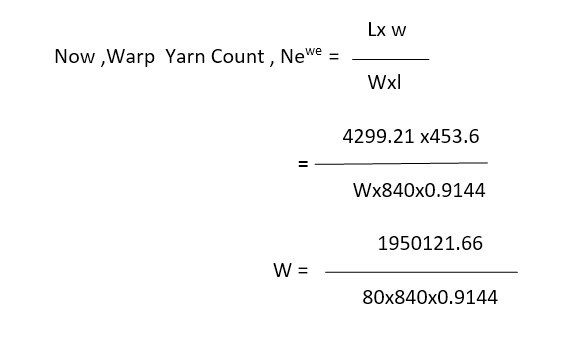=      31.74 gm

So the GSM of the fabric will be = 27.54 + 31.74

= 59.28

Here is a screenshot of a GSM calculator giving the same input and the result is correct !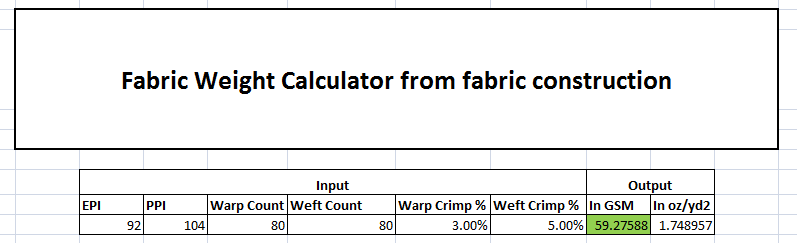(47236)#### Taukir Kabir Tusar